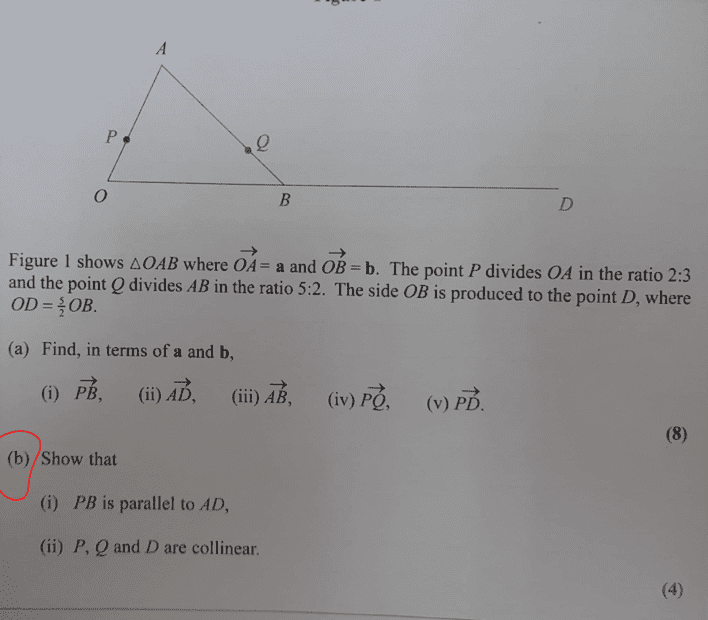# Show that P, Q and D are collinear in the vector problem

Gold Member
Homework Statement:
See attached
Relevant Equations:
VectorsMy interest is only on part (b),

For part (i), My approach is as follows,
##PB=PO+OB##
##PB=-\dfrac{2}{5}a +b##

For part (ii), we shall have;

##QD=QB+BD##

##QD=\dfrac{2}{7}AB+\dfrac{3}{2}b##

##QD=-\dfrac{2}{7}a+\dfrac{2}{7}b+\dfrac{3}{2}b##

##QD=\dfrac{25}{14}b-\dfrac{2}{7}a##

also,
##PQ=PA+AQ##

##PQ=\dfrac{3}{5}a-\dfrac{5}{7}a+\dfrac{5}{7}b##

##PQ=\dfrac{5}{7}b-\dfrac{4}{35}a##

Therefore,

##PQ=\dfrac{1}{7}\left[5b-\dfrac{4}{5}a\right]##
&
##QD=\dfrac{1}{7}\left[\dfrac{25}{2}b-2a\right]##

It follows that,

##PQ=\dfrac{5}{7}\left[25b-4a\right]##

##QD=\dfrac{2}{7}\left[25b-4a\right]##

##OQ=\dfrac{2}{5} PQ## thus shown as there is a scalar connecting the two...seeking any alternative approach and i hope my working is correct as i do not have solutions to these questions...

Last edited:
•Delta2

Homework Helper
Gold Member
for (b) (i) I find it correct
for (b) (ii) there seem to be some typos after the phrase "It follows that" please correct them. For example I think it should $$\vec{PQ}=\frac{1}{5\cdot 7}[25\vec{b}-4\vec{a}]$$ and the final equation should involve PQ and QD not OQ(?)

Last edited:
Gold Member
for (b) (i) I find it correct
for (b) (ii) there seem to be some typos after the phrase "It follows that" please correct them. For example I think it should $$\vec{PQ}=\frac{1}{5\cdot 7}[25b-4a]$$ and the final equation should involve PQ and QD not OQ(?)
Ok...I will check on that ...

•Delta2
Homework Helper
Gold Member
The correct final equation should be ##\vec{PQ}=\frac{2}{5}\vec{QD}##, at least that's what i think.

•chwala
Gold Member
The correct final equation should be ##\vec{PQ}=\frac{2}{5}\vec{QD}##, at least that's what i think.
That should be correct...my working is fine, but the division part at the end was wrong...

•Delta2
Gold Member
Homework Statement:: See attached
Relevant Equations:: Vectors

View attachment 301993

My interest is only on part (b),

For part (i), My approach is as follows,
##PB=PO+OB##
##PB=-\dfrac{2}{5}a +b##

For part (ii), we shall have;

##QD=QB+BD##

##QD=\dfrac{2}{7}AB+\dfrac{3}{2}b##

##QD=-\dfrac{2}{7}a+\dfrac{2}{7}b+\dfrac{3}{2}b##

##QD=\dfrac{25}{14}b-\dfrac{2}{7}a##

also,
##PQ=PA+AQ##

##PQ=\dfrac{3}{5}a-\dfrac{5}{7}a+\dfrac{5}{7}b##

##PQ=\dfrac{5}{7}b-\dfrac{4}{35}a##

Therefore,

##PQ=\dfrac{1}{7}\left[5b-\dfrac{4}{5}a\right]##
&
##QD=\dfrac{1}{7}\left[\dfrac{25}{2}b-2a\right]##

It follows that,

##PQ=\dfrac{5}{7}\left[25b-4a\right]##

##QD=\dfrac{2}{7}\left[25b-4a\right]##

##QD=\dfrac{2}{5} PQ## thus shown as there is a scalar connecting the two...seeking any alternative approach and i hope my working is correct as i do not have solutions to these questions...
Amended to ##QD##...I will amend division part later, using my android to type...

Homework Helper
Gold Member
I find the expressions of PQ and QD wrong after the phrase "It follows that" regarding the multiplicative constant of each, please review and try to correct them.

Gold Member
I find the expressions of PQ and QD wrong after the phrase "It follows that" regarding the multiplicative constant of each, please review and try to correct them.
Yes I will...thanks for your input...

•Delta2
Gold Member
Homework Statement:: See attached
Relevant Equations:: Vectors

View attachment 301993

My interest is only on part (b),

For part (i), My approach is as follows,
##PB=PO+OB##
##PB=-\dfrac{2}{5}a +b##

For part (ii), we shall have;

##QD=QB+BD##

##QD=\dfrac{2}{7}AB+\dfrac{3}{2}b##

##QD=-\dfrac{2}{7}a+\dfrac{2}{7}b+\dfrac{3}{2}b##

##QD=\dfrac{25}{14}b-\dfrac{2}{7}a##

also,
##PQ=PA+AQ##

##PQ=\dfrac{3}{5}a-\dfrac{5}{7}a+\dfrac{5}{7}b##

##PQ=\dfrac{5}{7}b-\dfrac{4}{35}a##

Therefore,

##PQ=\dfrac{1}{7}\left[5b-\dfrac{4}{5}a\right]##
&
##QD=\dfrac{1}{7}\left[\dfrac{25}{2}b-2a\right]##

It follows that,

##PQ=\dfrac{5}{7}\left[25b-4a\right]##

##QD=\dfrac{2}{7}\left[25b-4a\right]##

##OQ=\dfrac{2}{5} PQ## thus shown as there is a scalar connecting the two...seeking any alternative approach and i hope my working is correct as i do not have solutions to these questions...
We ought to have;

It follows that,

##PQ=\dfrac{1}{35}\left[25b-4a\right]##

##QD=\dfrac{1}{14}\left[25b-4a\right]##

##35 PQ=(25b-4a)##
##14QD=(25b-4a)##

##35PQ=14QD##
##PQ=\dfrac{14}{35} QD##
##PQ=\dfrac{2}{5} QD##

•Delta2